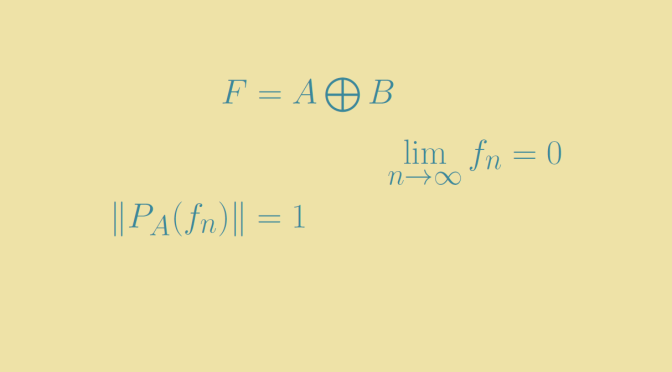# Two algebraically complemented subspaces that are not topologically complemented

We give here an example of a two complemented subspaces $$A$$ and $$B$$ that are not topologically complemented.

For this, we consider a vector space of infinite dimension equipped with an inner product. We also suppose that $$E$$ is separable. Hence, $$E$$ has an orthonormal basis $$(e_n)_{n \in \mathbb N}$$.

Let $$a_n=e_{2n}$$ and $$b_n=e_{2n}+\frac{1}{2n+1} e_{2n+1}$$. We denote $$A$$ and $$B$$ the closures of the linear subspaces generated by the vectors $$(a_n)$$ and $$(b_n)$$ respectively. We consider $$F=A+B$$ and prove that $$A$$ and $$B$$ are complemented subspaces in $$F$$, but not topologically complemented.

### $$A$$ and $$B$$ are complemented subspaces in $$F$$

Let $$\langle \cdot , \cdot \rangle$$ be the inner product. For $$i \neq j$$ integers we have $$\langle a_i, a_j \rangle =0$$ and $$\langle b_i, b_j \rangle =0$$ as $$(e_n)_{n \in \mathbb N}$$ is an orthonormal basis. Therefore all $$x \in A$$ can be written $$\displaystyle \sum_{n=0}^{+\infty} \langle x , e_{2n} \rangle e_{2n}$$ and all $$x \in B$$ can be written $$\displaystyle \sum_{n=0}^{+\infty} \langle x , b_n \rangle \frac{b_n}{\Vert b_n \Vert^2}$$.

If $$x \in A \cap B$$, we have for all $$n \in \mathbb N$$ $$\langle x, e_{2n+1} \rangle =0$$ as $$x \in A$$. Hence $x=\sum_{n=0}^{+\infty} \langle x , e_{2n} \rangle \frac{b_n}{\Vert b_n \Vert^2}$ Making the inner product of $$x$$ with $$e_{2n+1}$$ we get $\langle x, e_{2n+1} \rangle = \langle x , e_{2n} \rangle \frac{\Vert e_{2n+1} \Vert^2}{(2n+1)\Vert b_n \Vert^2} = 0$ Consequently, we have $$\langle x, e_{2n} \rangle$$ for all $$n \in \mathbb N$$ and finally $$x = 0$$ which proves that $$A$$ and $$B$$ are complemented subspaces in $$F$$.

### $$A$$ and $$B$$ are not topologically complemented

Let $$(x_n)$$ be the sequence $$x_n = b_n-a_n$$. We have $$x_n=\frac{e_{2n+1}}{n+1}$$ and $$\lim\limits_{n \to \infty} x_n = 0$$. If $$A$$ and $$B$$ were topologically complemented, the projection of $$F$$ on the subspace $$A$$ would be continuous. But the projection of $$x_n$$ is $$a_n$$ as $$A$$ and $$B$$ are complemented subspaces. However $$\Vert a_n \Vert = 1$$ for all $$n \in \mathbb N$$ as $$(e_n)$$ is an orthonormal basis. A contradiction proving that $$A$$ and $$B$$ are not topologically complemented.